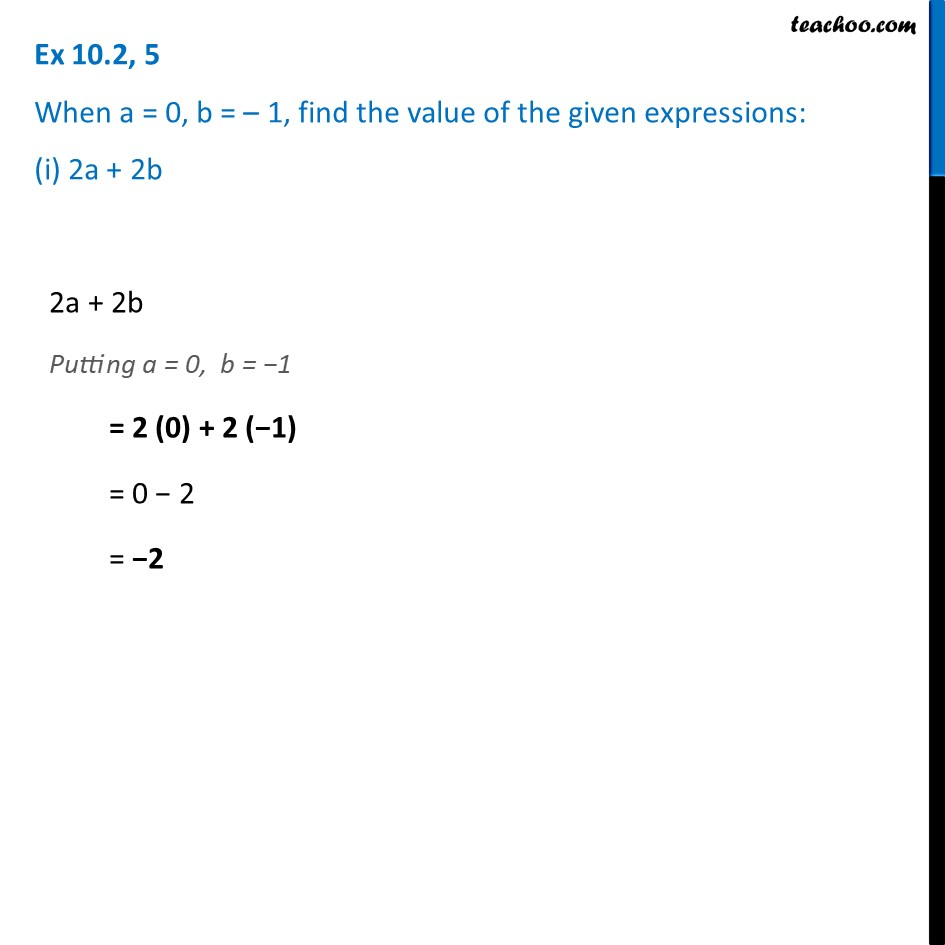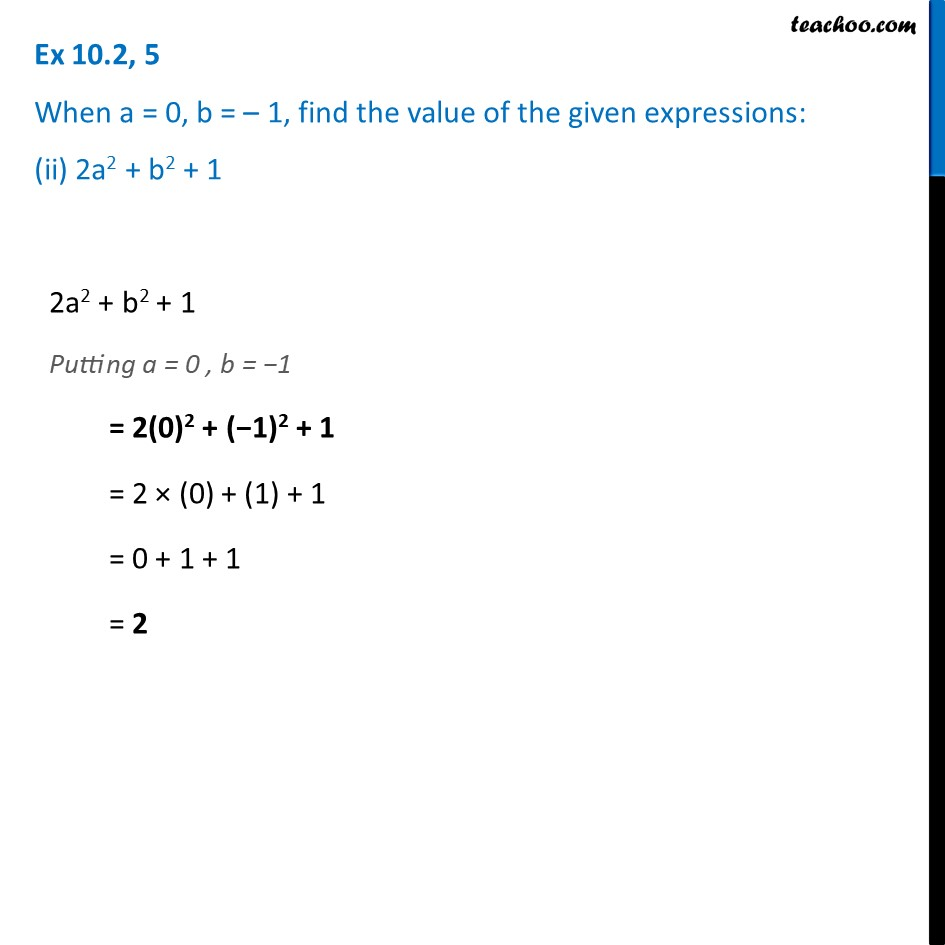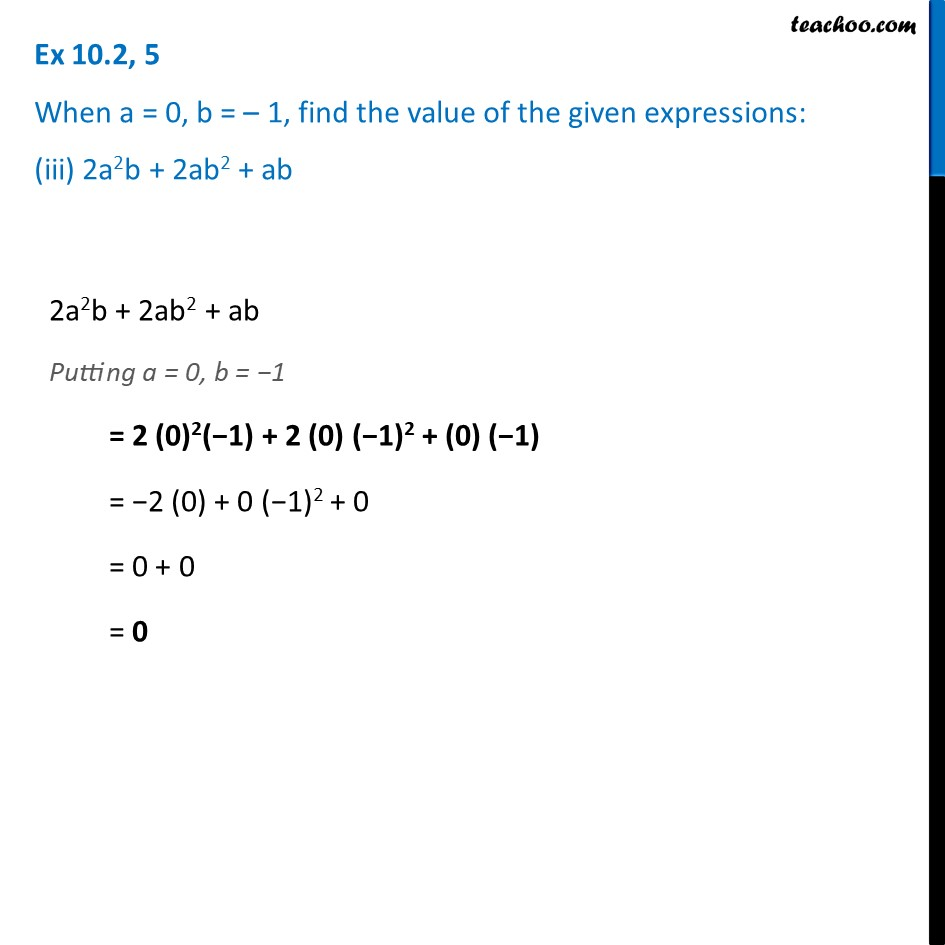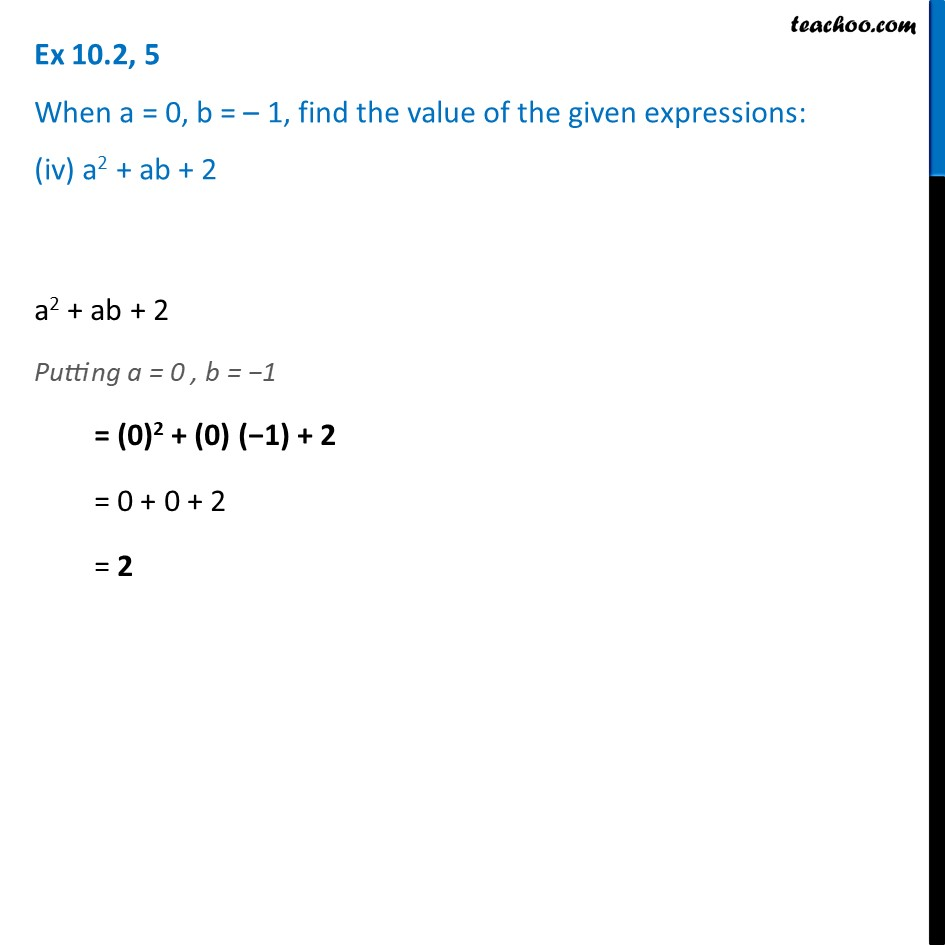Ex 10.2

Chapter 10 Class 7 Algebraic Expressions
Serial order wiseLearn in your speed, with individual attention - Teachoo Maths 1-on-1 Class

### Transcript

Ex 10.2, 5 When a = 0, b = – 1, find the value of the given expressions: (i) 2a + 2b 2a + 2b Putting a = 0, b = −1 = 2 (0) + 2 (−1) = 0 − 2 = −2 Ex 10.2, 5 When a = 0, b = – 1, find the value of the given expressions: (ii) 2a2 + b2 + 1 2a2 + b2 + 1 Putting a = 0 , b = −1 = 2(0)2 + (−1)2 + 1 = 2 × (0) + (1) + 1 = 0 + 1 + 1 = 2 Ex 10.2, 5 When a = 0, b = – 1, find the value of the given expressions: (iii) 2a2b + 2ab2 + ab 2a2b + 2ab2 + ab Putting a = 0, b = −1 = 2 (0)2(−1) + 2 (0) (−1)2 + (0) (−1) = −2 (0) + 0 (−1)2 + 0 = 0 + 0 = 0 Ex 10.2, 5 When a = 0, b = – 1, find the value of the given expressions: (iv) a2 + ab + 2a2 + ab + 2 Putting a = 0 , b = −1 = (0)2 + (0) (−1) + 2 = 0 + 0 + 2 = 2